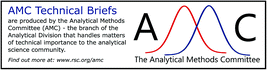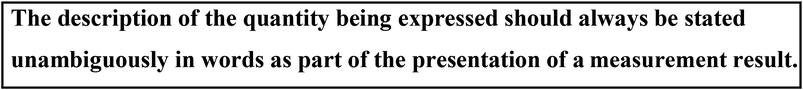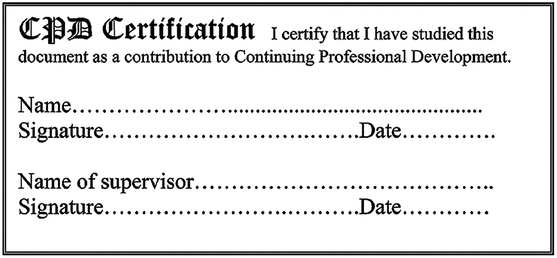# Units and quantities for analytical chemistry (background paper)

Analytical Methods Committee, AMCTB No. 99

First published on 2nd October 2020

It is universally accepted that a measurement of a quantity of interest should be presented as the product of a numerical value and a unit (we should also include an estimate of measurement uncertainty, but this is beyond the scope of this Technical Brief). The International System of Units (the SI) provides the world’s only practical system of coherent units of measurement for this purpose. For ease of expression and understanding it is generally preferred that the numerical value presented is between 1 and 100. One might refer to these as ‘human-scale’ numbers that are easy to relate to, conceptualise and communicate. The SI uses ‘SI prefixes’ to achieve this aim, allowing us to write 2.3 km rather than 2300 m. Equally we could use scientific nomenclature to write, arguably more inelegantly, 2.3 × 103 m. Deviation from these agreed practices may risk confusion in the communication of measurement results. This Technical Brief outlines the SI units and quantities available for use in analytical chemistry and explains the care that must be taken if alternative approaches are followed. One principle remains essential to aid understanding: the description of the quantity being expressed should always be stated unambiguously in words as part of the presentation of a measurement result.

Analytical chemistry is largely concerned with determining the composition of mixtures. The result of analysis for a component in a mixture should comprise a ‘numerical value’ and a ‘unit’ in order to express the value of the ‘quantity’ being measured (and an associated statement of uncertainty, of course1). The International System of Units (the SI) provides the world’s only practical system for this.2 ‘Amount of substance’ (often shortened to ‘amount’), the quantity used to characterise a number of (chemical) entities, is rarely measured directly in analytical chemistry. In practice, the amount, mass or volume of a substance (all extensive quantities – those whose magnitude is proportional to the size of the system described) is usually combined with the amount, mass, or volume of the entire mixture (more extensive quantities), to derive quantities that express the composition of mixtures (all intensive quantities – those whose magnitude is independent of the size of the system described).3,4 These quantities are subtly different, so it is helpful to clarify the advantages and drawbacks of using each type.## Quantities

Table 1 lists nine quantities that are commonly used to characterise the composition of mixtures. Although these quantities are related by simple factors, such as density and relative molecular mass, there are important differences in precisely how they define composition. The quantity mass fraction has the property that it can be used to describe the composition of a component in a mixture when only knowledge of the total mass of the sample and the mass of the component of interest are available. Amount content and molality (amount of the component of interest divided by the mass of the solvent) require this information together with the identity of the component being described and its molar mass. The use of these quantities does not require detailed knowledge of the composition of other components within the mixture, nor are they sensitive to temperature or pressure. Therefore, these quantities are the most suitable for routine use in the description of the presence of an analyte of interest in an otherwise uncharacterised sample. In contrast, quantities involving amount as a denominator require an exact knowledge of all components within the mixture and are best used when the mixture is fully characterised, for instance a calibration standard prepared by an analyst from materials of known purity.
Table 1 Quantities used to characterise composition. The meaning of the symbols in the table is as follows: mi, Vi, and ni are the mass, volume (prior to mixing), and the amount, of component i; ∑mj, ∑Vj and ∑nj are the sums of the masses, volumes (prior to mixing), and the amounts, of all components; msolv is the mass of the solvent only; m and V are the total mass, and total volume (after mixing), of the mixture. Fractions describe how much of the total property of a sample is contributed by one of its constituent substances; concentrations describe the ratio of one extensive quantity of a single substance to the total volume of the mixture; and contents describe the ratio of one extensive quantity of a substance to the total mass of the mixture. Molality describes the amount of solute entities divided by the mass of the solvent. Adapted from ref. 3 and 8
Quantity Symbol Definition SI unit Other common units
Mass fraction w wi = mi/∑mj kg kg−1 g g−1, μg g−1, ng g−1, mg kg−1, μg kg−1, etc.
Volume fraction ϕ ϕi = Vi/∑Vj m3 m−3 L L−1, mL L−1, etc.
Amount fraction x xi = ni/∑nj mol mol−1 mmol mol−1, μmol mol−1, nmol mol−1, etc.
Mass concentration γ γi = mi/V kg m−3 g dm−3, g L−1, mg dm−3, mg L−1, etc.
Volume concentration σ σi = Vi/V m3 m−3 L L−1, mL L−1, etc.
Amount concentration (molarity) c ci = ni/V mol m−3 M, mol dm−3, mol L−1, mM, mmol dm−3, mmol L−1, etc.
Molality b bi = ni/msolv mol kg−1 mmol kg−1, μmol kg−1, etc.
Volume content κ κi = Vi/m m3 kg−1 cm3 g−1, etc.
Amount content k ki = ni/m mol kg−1 mmol kg−1, μmol kg−1, etc.

Quantities involving volumes are more limited in their application because their magnitude is dependent on temperature, and pressure (for gaseous mixtures), and therefore for mass concentrations and amount concentrations it is often necessary to state at what temperature and pressure the value of the quantity is applicable. Indeed the use of volume fraction and volume concentration is discouraged without reiteration of what is being expressed, since definitions vary as to whether the volume of the whole mixture is measured before or after the mixing of individual components. Only when discussing the general concept, and there is no risk of any confusion, should the term ‘concentration’ be used in isolation, perhaps because the quantity being expressed has already been described in detail.

## Units

Table 1 shows the SI units in common use when describing chemical composition. Since the units used for some of these quantities such as amount fraction, mass fraction and volume fraction are mol mol−1, kg kg−1, and m3 m−3, respectively, they are often referred to as dimensionless quantities or, in the current SI Brochure,5 as quantities with the unit one. This is because the expression for their units can be simplified to one (i.e. mol mol−1 = 1). The terms ‘parts per million (ppm)’, ‘parts per billion (ppb)’, ‘parts per trillion (ppt)’, ‘per mille (‰)’ and ‘per cent (%)’ are often used in place of units to express mass, volume or amount fractions. These terms are not strictly units but represent instead the numerical multipliers 10−6, 10−9, 10−3 and 10−2.6 Other variations of these terms in common use, such as % (v/v), % (m/m), ppmv, ppbv, wt%, w/w%, vol%, w/v%, atom%, mol%, etc., are best avoided because their meanings are considerably less well understood and standardised, and moreover they violate the general principle that the description of a quantity should not be mixed with the presentation of a unit. For instance, rather than present a mass fraction measurement (symbol w) as w = 0.5% (m/m), we should write instead w = 0.5%, or even better w = 5 g/kg.

The use of ‘ppm’ and ‘ppb’ and similar terms is widespread in analytical chemistry, in particular when proper units are omitted because the quantity being expressed is a fraction where the units can be simplified to one. When these terms are used it is essential that a full description of the quantity being measured is provided so there can be no ambiguity in the interpretation of the result of a measurement (since ‘ppm’ could represent any type of fraction) and that the meaning of the terms used is explained (for instance there are different meanings for ‘billion’, ‘trillion’, etc., across the world).7 The use of ‘ppm’ and ‘ppb’ in specific situations may afford some advantages: used to express amount fraction they are the main route to document workplace exposure limits across the world and so usually involve more ‘rounded’, memorable values than the equivalent numerical value expressed as a mass concentration in mg m−3. However, whenever possible the terms ‘ppm’ and ‘ppb’ should not be used to express concentrations. By its definition (in Table 1) a concentration does not have units that simplify to one (except for the rather obscure quantity volume concentration). The use of ‘ppm’ and ‘ppb’ might also allow otherwise complicated unit expressions to be simplified. For instance uptake rate of gaseous diffusive samplers expressed in (ng ppm−1) min−1 rather than (ng (μmol mol−1)−1) min−1.## Expressing the result of a measurement

Ideally the description of the quantity being expressed should always be stated unambiguously in words as part of the presentation of a result. This provides clarity and avoids confusion (especially between quantities such as molality and amount content, and between mass fraction and amount fraction). It is best practice for amount, mass, and volume fractions, as dimensionless quantities, to use explicit units to avoid confusion and express the maximum information about the quantity being expressed. For example, it is best to state that:

• The amount fraction of calcium, x(Ca) = 2.3 × 10−6 mol mol−1, or

• The amount fraction of calcium, x(Ca) = 2.3 μmol mol−1.

It is arguably less rigorous to state that:

• The amount fraction of calcium, x(Ca) = 2.3 × 10−6, or

• The amount fraction of calcium, x(Ca) = 2.3 ppm.

And it is ambiguous to state that:

x(Ca) = 2.3 ppm.

In the final example, the use of ‘ppm’ does not unambiguously distinguish between quantities such as mass fraction, amount fraction, volume fraction and volume concentration. The reader is left to guess whether the symbol x has been employed to represent the quantity amount fraction, or not.

This Technical Brief has presented, from the viewpoint of the SI, the internationally agreed units and quantities available to express measurement results in analytical chemistry, with best practice guidelines suggested – noting that some units must be used with care since their numerical values are sensitive to environmental conditions. There are also other expressions that are in common usage and the Technical Brief has explained the care that must be taken to avoid confusion when employing these alternatives. In all cases, the key principle that must be followed to ensure unambiguous communication is to state clearly in words the description of the quantity being expressed as part of the presentation of a measurement result.

Dr Richard J. C. Brown (National Physical Laboratory)

This Technical Brief was prepared for the Analytical Methods Committee, with contributions from Michael Healy (Environment Agency), Matthew Rawlinson (Affinity Water Ltd) and Ian Pengelly (Health and Safety Executive), and approved on 31st August 2020.1. EURACHEM/CITAC Guide CG 4, Quantifying Uncertainty in Analytical Measurement, ed. S. L. R. Ellison and A. Williams, EURACHEM/CITAC, 3rd edn, 2012 Search PubMed.
2. AMC TB, Revision of the International System of Units, Anal. Methods, 2019, 11, 1577–1579 RSC.
3. T. Cvitas, Quantities describing compositions of mixtures, Metrologia, 1996, 33, 35–39 CrossRef.
4. Quantities Units and Symbols in Physical Chemistry, ed. I. Mills, et al., RSC Publishing, Cambridge, 3rd edn, 2007 Search PubMed.
5. The International System of Units, BIPM, 9th edn, 2019, https://www.bipm.org/en/publications/si-brochure/ Search PubMed.
6. T. J. Quinn and I. M. Mills, The use and abuse of the terms percent, parts per million and parts in 10⁁n, Metrologia, 1998, 35, 807–810 CrossRef.
7. Long and short scales, https://en.wikipedia.org/wiki/Long_and_short_scales, accessed June 2020 Search PubMed.
8. R. J. C. Brown, Quantities and units in analytical chemistry, Int. J. Environ. Anal. Chem., 2008, 88, 681–687 CrossRef CAS.

### Footnotes

Each of the seven base quantities used in the SI (mass, length, time, amount of substance, etc.) is regarded as having its own independent dimension. Derived quantities written in terms of the base quantities, whose dimensions cannot be simplified to one, are quantities with dimension, e.g. velocity in m s−1, area in m2, etc.
The unit here has been expressed as a quotient, as in g/kg, but could equally have been expressed as products using negative exponents, as in g · kg−1 or g kg−1, as in the rest of the paper.4
 This journal is © The Royal Society of Chemistry 2020